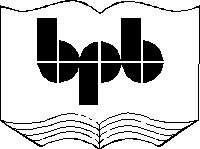2

Conditions and Loops

In this chapter, we shall discover the conditional statements and the loops that are provided for, in WMLScript. These are the very basic components of every programming language.

· Conditions enable a programmer to conditionally execute or prevent execution of certain parts of the code.

· Loops provide a mechanism whereby, a specific piece of code is executed repeatedly, either for a specified number of iterations or until some condition is satisfied.

These two mechanisms render the software program with the requisite intelligence. So far, we have only shown you code that is static in behaviour and does not fall in the realm of things dynamic. This is because, every statement in the code is executed sequentially and executed only once. We intend to rectify this situation using conditions and loops.

The WML file looks as follows :

a11.wml

<?xml version="1.0"?>

<!DOCTYPE wml PUBLIC "-//WAPFORUM//DTD WML 1.1//EN" "http://www.wapforum.org/DTD/wml_1.1.xml">

<wml>

<card newcontext="true">

<do type="xx" >

<go href="aaa.wmls#abc()">

</go>

</do>

<p>

Hello \$aa

</p>

</card>

</wml>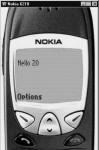Screen 2. 1

aaa.wmls

extern function abc()

{

var yy;

yy = 10;

if ( true)

yy = 20;

WMLBrowser.setVar("aa",yy);

WMLBrowser.refresh();

}

In this example, we have a variable called yy and we are assigning it a value of 10. The next statement is if(true). The if statement is passed either a true or a false value. True means YES, while false means NO. Since we have used if(true), the next line gets executed. Therefore, the value of yy becomes 20 and hence, aa gets displayed as 20.

You are bound to wonder as to what is so original about this program. The if statement could simply be removed and everything else would work as normal. So, to demonstrate the usefulness of the if conditional statement, we change the true to false. When we do so, the next line gets ignored. Thus, yy = 20 doesn't get displayed at all.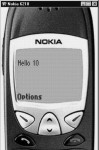Screen 2. 2

aaa.wmls

extern function abc()

{

var yy;

yy = 10;

if ( false)

yy = 20;

WMLBrowser.setVar("aa",yy);

WMLBrowser.refresh();

}

Using the if statement, we have prevented the execution of certain lines of code conditionally. The only restriction of the if statement so far is that, it acts only on the line that immediately succeeds it. So, the statement yy = 30 gets executed.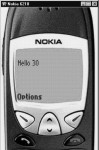Screen 2. 3

aaa.wmls

extern function abc()

{

var yy;

yy = 10;

if ( false)

yy = 20;

yy = 30;

WMLBrowser.setVar("aa",yy);

WMLBrowser.refresh();

}

If you want the if statement to act on multiple lines, then you have to enclose them within curly braces viz. {  and  }. This is depicted below.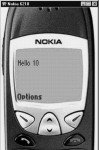Screen 2. 4

aaa.wmls

extern function abc()

{

var yy;

yy = 10;

if ( false)

{

yy = 20;

yy = 30;

}

WMLBrowser.setVar("aa",yy);

WMLBrowser.refresh();

}

In the above example, since if(false) has been used and the two succeeding lines are enclosed in curly braces, both these lines i.e. yy=20 and yy=30 do not get executed. So, how can we put this if statement to practical use? The mere use of true or false in the if  statement doesn't make sense. So, lets place conditions within the if statement. For example, we now say if(yy> 5).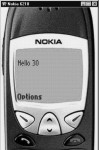Screen 2. 5

aaa.wmls

extern function abc()

{

var yy;

yy = 10;

if ( yy >5)

{

yy = 30;

}

WMLBrowser.setVar("aa",yy);

WMLBrowser.refresh();

}

If the value of the variable yy is greater than 5, the code y=30 is to be executed, otherwise not.

The Relational Operators available in WML are as follows :

=          assignment operator

>          greater than

<          less than

>=        greater than or equal to

<=        less than or equal to

!=         not equal to

==        equal to

These operators determine if the given condition is true or false.Screen 2. 6

aaa.wmls

extern function abc()

{

var yy;

yy = 10;

if ( yy > 5)    //if ( yy >= 5)

{

yy = 30;

}

WMLBrowser.setVar("aa",yy);

WMLBrowser.refresh();

}

aaa.wmls

extern function abc()

{

var yy;

yy = 1;

if ( yy < 5)        //if ( yy <= 5)

{

yy = 30;

}

WMLBrowser.setVar("aa",yy);

WMLBrowser.refresh();

}

aaa.wmls

extern function abc()

{

var yy;

yy = 5;

if ( yy == 5)

{

yy = 30;

}

WMLBrowser.setVar("aa",yy);

WMLBrowser.refresh();

}

aaa.wmls

extern function abc()

{

var yy;

yy = 0;

if ( yy != 5)

{

yy = 30;

}

WMLBrowser.setVar("aa",yy);

WMLBrowser.refresh();

}

Let us take a case where you have an input box and in that input box, the user inputs the value 100. After he clicks on OK, your WMLscript gets called. In the script, you can have an if statement that scrutinizes whether the inputted value is within a specified range or not. If it is so, then some specified code is executed; and if not, then some other statements are executed.

You may want to exhibit something to the younger bunch of people, and at the same time, you may not want to divulge it to the older lot. This is where the if statement gives you the flexibility to do so.

The if statement also has an else statement associated with it. So, when the if statement evaluates to true,  the statements within the open and close of the brackets immediately following the if statement, get executed. Otherwise, the statements following the else get executed.

Now, here is an example that uses the if and the else statements.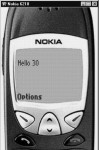Screen 2. 7

aaa.wmls

extern function abc()

{

var yy;

yy = 10;

if ( yy< 50)

{

yy = 30;

}

else

{

yy = 70;

}

WMLBrowser.setVar("aa",yy);

WMLBrowser.refresh();

}

In the above case, you need not have used the combination of the if and the else statements. Instead, you could have said if (yy < 50). And, in the next statement you could have said if (yy <= 50). At this juncture, the utility of the if and else constructs may not be very apparent. You will be able to appreciate it better when you look at some more examples subsequently.

The if statement has been provided for rendering intelligence to the language. You can nest one if statement within another if statement. Further, you can have as many levels of nesting of the if statements within each other, as you want.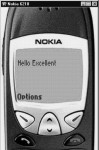Screen 2. 8

aaa.wmls

extern function abc()

{

var yy,zz;

yy = 80;

if ( yy > 50)

{

if (yy >=70 )

zz="Good" ;

if (yy >=80 )

zz="Excellent" ;

}

WMLBrowser.setVar("aa",zz);

WMLBrowser.refresh();

}

We had mentioned earlier that the WMLBrowser is a free library. There is another free library called Dialogs. If you use Dialogs.alert and pass "hi" as a parameter, you will see a bold  "hi" displayed on the left side of your screen. When you click on OK button, the program terminates.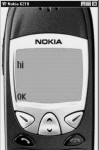Screen 2. 9

aaa.wmls

extern function abc()

{

}

You can attempt one more example. Use Dialogs.alert("hi"), and  then use Dialogs.alert("bye"). You will first see "hi" displayed on the screen. When you click on the OK button, the word "bye" will be displayed.

aaa.wmls

extern function abc()

{

}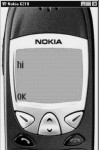Screen 2. 10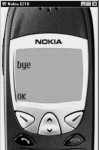Screen 2. 11

So, this is one way of displaying multiple screens. When the program starts, it displays a particular screen and when you click on the OK button, the program shows you another screen. While programming in Windows, this Dialogs.alert is called a MessageBox.

If we want to display the value of a variable in an alert, there will be a problem if it is a numeric value. This is so because the alert statement expects a string and not a number. So, we have to use one more library called String which has a function called format. In this function, we have to say Hello %d . Corresponding to the %d, we have to supply the variable, which is bb in the current example. Here, bb has a value of 20. So, the string has the value of "Hello 20". This now becomes the return value which will be stored in aa. So aa now contains the string "Hello 20".  This is how functions return a value.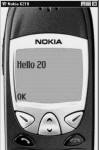Screen 2. 12

aaa.wmls

extern function abc()

{

var aa,bb ;

bb = 20;

aa = String.format("Hello %d",bb);

}

Similar to the function setVar, there is a complementary function called getVar, which returns a value that can be stored in another variable. This is another way of creating a string at runtime which has the value of a variable.

Having seen the if conditional statements, let us explore the concept of loops. Looping means repetition.

aaa.wmls

extern function abc()

{

var aa,bb ;

for ( bb = 1 ; bb <= 4; bb = bb + 1)

{

aa = String.format("Hello %d",bb);

}

}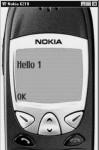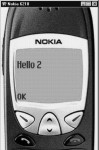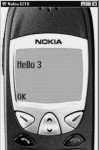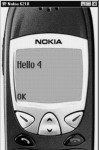Screen 2. 13 Screen 2. 14 Screen 2. 15 Screen 2. 16

In this script, we have created two variables, viz. aa and bb.  Then, we have used a for statement. The syntax of the for statement requires two semicolons. The statement before the first semicolon will be executed only once. The variable bb is assigned the value of 1. After the first semicolon, we have placed the conditional statement which says bb <= 4. Here, the for loop checks whether the condition    bb <= 4 is TRUE or not. If it is TRUE, it will execute the statements between the open and close curly braces. Within the curly braces, we have the statement String.format (Hello %d,bb). Since the value of bb is 1, "Hello 1" is displayed.  When we click on the OK button of the Dialogs.alert, the value of bb now becomes 2. Since the condition 2 <= 4 is true, "Hello 2" is displayed. Similarly, "Hello 3" and "Hello 4" are displayed. When bb becomes 5, the for condition becomes false and the program goes out of the for loop. What we have achieved is the repetitive execution of the code within the curly braces of the for loop till a specified condition remains TRUE.

Instead of a for loop, a while loop can also be used.  The only difference is that the for loop syntax has two  semicolons which separate the initialization, condition and then the incrementing expression; whereas the while loop only has the condition within the brackets. The initialization takes place before entering the while loop, and the incrementing of the variable is done within the while loop.

aaa.wmls

extern function abc()

{

var aa,bb ;

bb = 1;

while  (bb <= 4   )

{

aa = String.format("Hello %d",bb);

bb = bb + 1;

}

}

Many programmers face a dilemma of using either a while or a for loop. There is absolutely no difference between the two because, whatever you use, when the code is finally converted into machine language, there is no difference in their bytecode.

aaa.wmls

extern function abc()

{

var aa,bb ;

aa = Dialogs.prompt("Hi","Bye");

}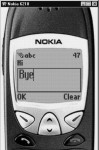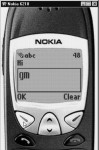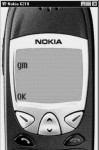Screen 2. 17 Screen 2. 18 Screen 2. 19

The Dialogs library has many useful functions. Dialogs.prompt is one of them. In the above example, the "hi" is displayed as a label on top and the "bye" is the default value. Whatever value is input by the  user, is assigned to the variable aa. This is the value that is displayed. This is the mechanism for accepting inputs from the user.

Dialogs.confirm is the next function that we shall see. You can ask the user as to what he wishes to do.  "Hi" is displayed in the first column, while "Bye" is displayed in the second column.  If the user clicks on "Bye", because it becomes TRUE, he sees an OK; whereas, if he clicks on No, the value becomes FALSE.

aaa.wmls

extern function abc()

{

var aa,bb ;

aa = Dialogs.confirm("Hi","Bye","No");

if (aa)

else

}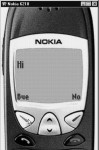Screen 2. 20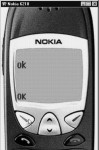Screen 2. 21

In the next script, we have a function called abc. In abc we call two functions, viz. pqr and xyz.

aaa.wmls

extern function abc()

{

pqr();

xyz();

}

function pqr()

{

}

function xyz()

{

pqr();

}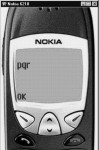Screen 2. 22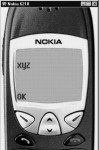Screen 2. 23

Whether you put the code of pqr below or above abc, is of no consequence. Further, since you do not need to call these functions from outside, you don't have to write the word extern. First, pqr gets called, which simply displays the dialog box with the letters "pqr". When the user clicks on OK, the function xyz gets called.  Then again, the letters "pqr" get displayed since the function prq is called by the function xyz.

You can call pqr six times and you can do so from anywhere. We have not used extern here because WML is not calling the function.  If the function pqr calls the function xyz, then, the program will not give any error message, but it will go into an indefinite loop.

Let us look at the next example.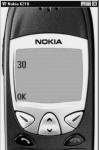Screen 2. 24

aaa.wmls

extern function abc()

{

var aa,i;

i = pqr(10,20);

aa = String.format("%d",i);

}

function pqr(p,q)

{

return p+q;

}

Function abc calls a function pqr.  It has two parameters named p and q. The variable p is assigned a value of 10 and q is assigned a value of 20. Then, we say return p+q. The return statement is used by functions to return values. When the return statement is executed, the variable i in abc will have the value of 30. This is the value that will get displayed.

In the following example, after the word return , we have put a Dialogs.alert that says "will not display".

aaa.wmls

extern function abc()

{

var aa,i;

i = pqr(10,20);

aa = String.format("%d",i);

}

function pqr(p,q)

{

return p+q;

}

Here, when the program reaches the return statement, the program returns. The execution of the program halts unconditionally and the lines of code following the return statement are not executed.

The next example shows that we have two variables called i and aa. We call a function called pqr and in pqr we create another variable called i. Both these variables are different.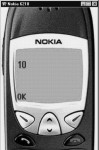Screen 2. 25

aaa.wmls

extern function abc()

{

var aa,i;

i = 10;

pqr(10,20);

aa = String.format("%d",i);

}

function pqr(p,q)

{

var i = 100;

}

The value of the variable i in abc is 10 and that of the variable i in pqr is 100. These variables are visible only within their functions.

In the next example we create a variable i in abc. When we say i=20 in pqr, we get an error. This is because we cannot access a variable created in one function in another function.

aaa.wmls

extern function abc()

{

var aa,i;

i = 10;

pqr(10,20);

aa = String.format("%d",i);

}

function pqr(p,q)

{

i = 100;

}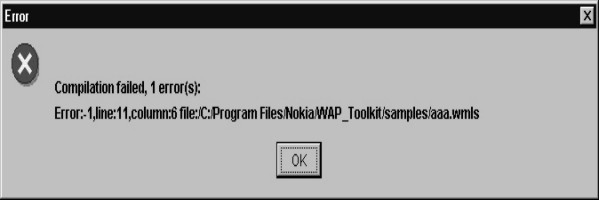Screen 2. 26

In next program, the function pqr has two parameters p and q. When we are say var p = 20 again, it results in an error, because passing parameters to a function is equivalent to using var at the start of the program. Therefore, you can't use var again.  The names have to be different. Further, if you have a variable in abc and a parameter in pqr, it is similar to using a var before starting the program.

aaa.wmls

extern function abc()

{

var aa,i;

i = 10;

pqr(10,20);

aa = String.format("%d",i);